# Absorption spectrum

• A
Hi everyone,
I have an absorption spectrum that I obtained from a sample after radiation and I need to use the background sample as a reference, how can I do that using excel?

what I did is subtract the radiated from the background spectrum then plot it, is that right?

Thanks.

mfb
Mentor
I would subtract the background from the signal not the opposite way, but anyway, that is just a sign.

If you don't run into a problem with statistical fluctuations that approach should be fine. More context would be useful, however.

mjc123
Homework Helper
To do a correct subtraction you should do it in absorbance mode, not transmittance; but perhaps you already knew that.

I subtracted the background from the signal but after plotting it seems that the points have spread!! why is that?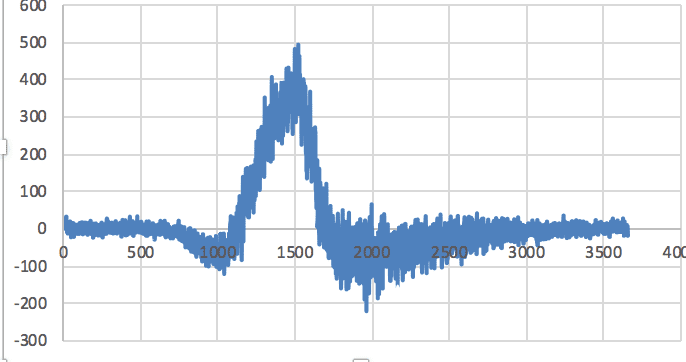then I tried to add them and the shape of the plot was looking more nicer, could someone explain what happened to me?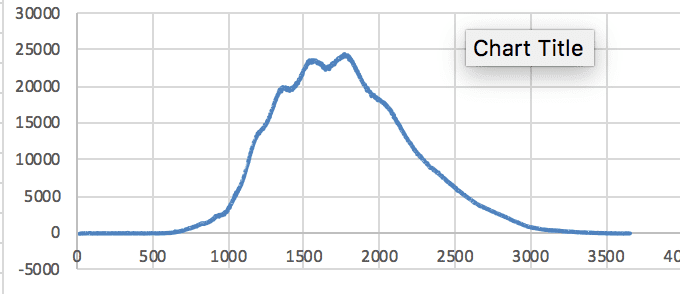#### Attachments

mjc123
Homework Helper
Can you show your sample and background spectra? It looks as if they are very similar, so the subtracted signal is much weaker than either spectrum. That's why the noise-to-signal ratio is much greater on your subtracted spectrum (I assume that's what you mean by the points "spreading").
What are your y axis units? If they are mAU, then your spectrum looks a bit too strong for accurate quantitative analysis. Can you repeat the experiment at a lower concentration?

Can you show your sample and background spectra? It looks as if they are very similar, so the subtracted signal is much weaker than either spectrum. That's why the noise-to-signal ratio is much greater on your subtracted spectrum (I assume that's what you mean by the points "spreading").
What are your y axis units? If they are mAU, then your spectrum looks a bit too strong for accurate quantitative analysis. Can you repeat the experiment at a lower concentration?
I added them to see the shape of the spectrum.
This is the spectra of the background and the first irradiated sample, respectively: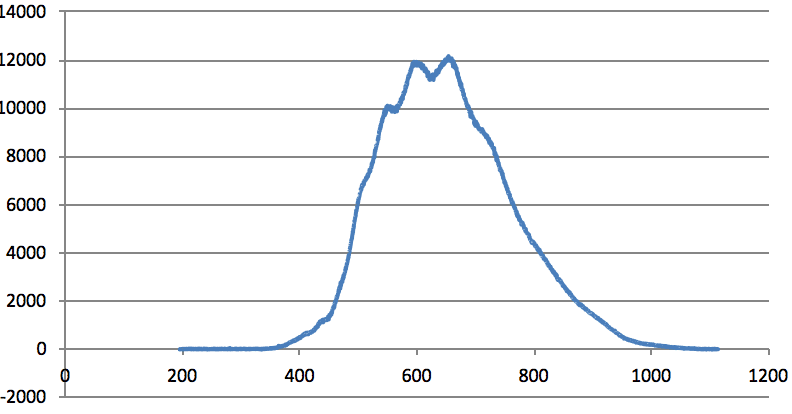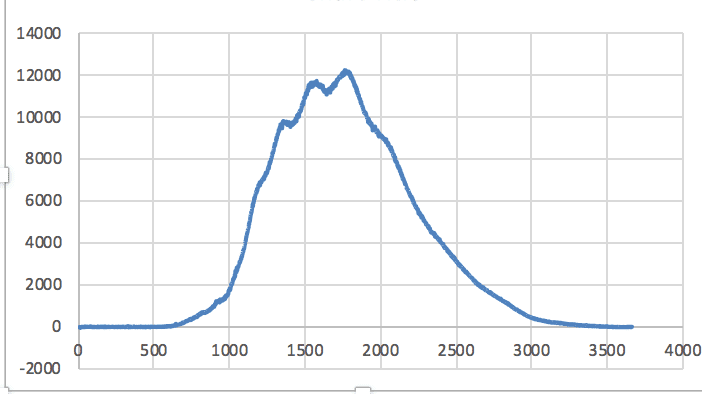As for the unit, I am not sure as it was my first time using that spectrometer, I am going to check and let you know.
If you mean the concentration of irradiated sample it is very law ( I am using FXO with Fe+2 (0.3mM) & XO (0.05mM)) all of them dissolved in 50 mM of H2SO4.

#### Attachments

Last edited:
mjc123
Homework Helper
What are your x-axis units here? You have very similar spectra, but one with the maximum at ca. 650 (nm? that would make sense) and the other at ca. 1800 (what? is it simply data point number in excel? I doubt very much if you scanned down to 0 nm!)
If you ran your spectra in a 10 mm cuvette, try using a 1 mm cuvette.
But I think you'll find it difficult getting a good subtraction spectrum. The difference between the spectra is of the same order of magnitude as the noise - as you found!

What are your x-axis units here? You have very similar spectra, but one with the maximum at ca. 650 (nm? that would make sense) and the other at ca. 1800 (what? is it simply data point number in excel? I doubt very much if you scanned down to 0 nm!)
If you ran your spectra in a 10 mm cuvette, try using a 1 mm cuvette.
But I think you'll find it difficult getting a good subtraction spectrum. The difference between the spectra is of the same order of magnitude as the noise - as you found!

The x-axis unite is nm. I tried to compare the 4th irradiated with the background
background spectra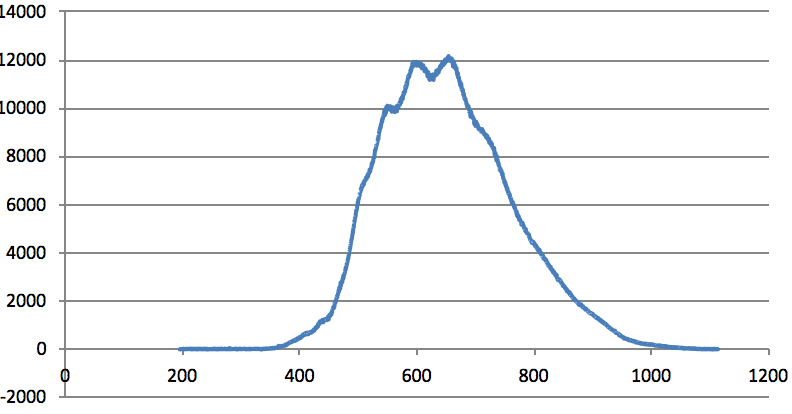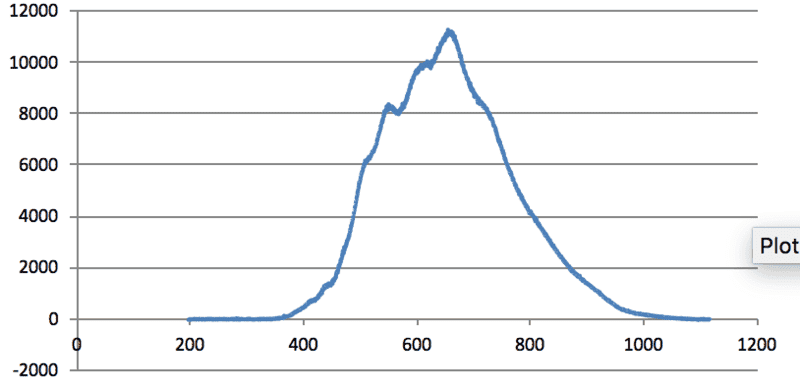subtraction of the above spectrums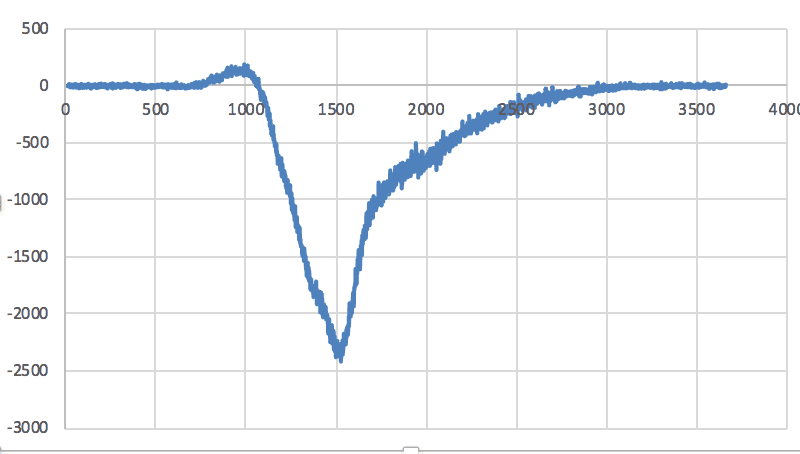Is it good that the spectra are in the minus y-axis or not, but I noticed that the points are not very spread which makes the noise less right?

#### Attachments

mjc123
Homework Helper
This is a bit better. You can see that the peak at 600 nm is reduced in the irradiated spectrum. This presumably corresponds to your negative peak at "1500" in the subtraction spectrum - get your x-axis units right. Yes, you should subtract the background from the irradiated spectrum, so your peak is negative.. Again, what do you mean by the points being "not very spread"?

This is a bit better. You can see that the peak at 600 nm is reduced in the irradiated spectrum. This presumably corresponds to your negative peak at "1500" in the subtraction spectrum - get your x-axis units right. Yes, you should subtract the background from the irradiated spectrum, so your peak is negative.. Again, what do you mean by the points being "not very spread"?
what do you mean to get my x-axis unit right?
In this picture, you can see that the points are very spread ( the spectra is not smooth)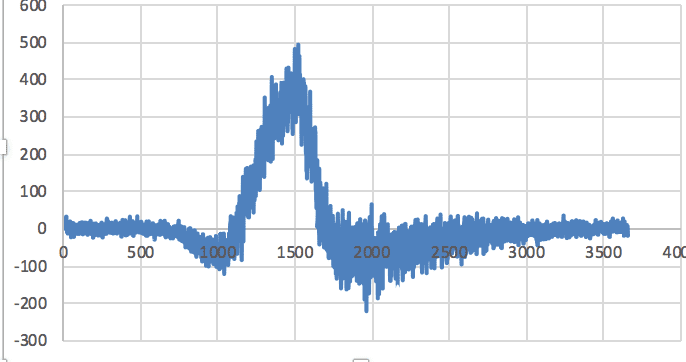but in the second one, it is smoother.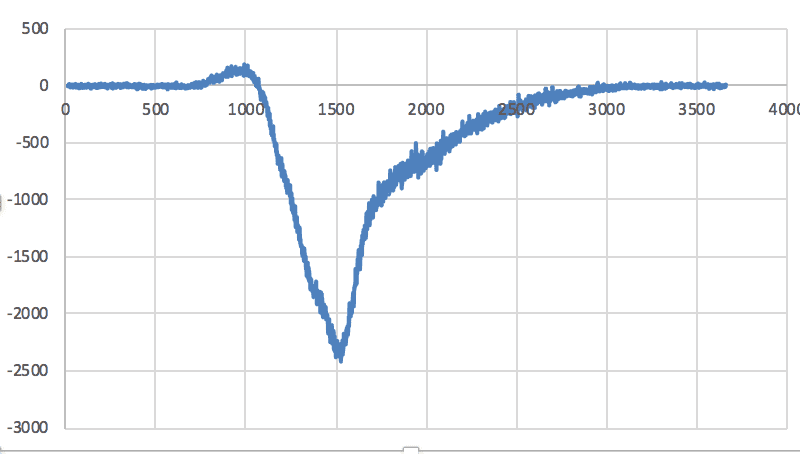#### Attachments

mfb
Mentor
what do you mean to get my x-axis unit right?
Your measured spectra are from 200 to 1100 nm. Why does the difference have x values of 0 to 3500?
In this picture, you can see that the points are very spread ( the spectra is not smooth)
That tells you that there is some noise in the measurement. Not surprising.
but in the second one, it is smoother.
The signal (the true difference) is stronger, so the signal to noise ratio is better.